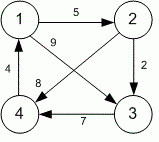Problems

# Floyd - 1

Time limit 1 second
Memory limit 128 MiB

The complete directed weighted graph is given with the weighted matrix. Construct a matrix of shortest paths between its vertices. It is guaranteed that the graph does not contain cycles of negative weight.

## Input data

The first line contains the number of vertices n~(1 \le n \le 100) in a graph. Each of the next n lines contains n numbers and describes the weighted matrix (the j-th number in the i-th row gives the weight of the edge from vertex i to vertex j). All the numbers by the absolute value do not exceed 100. The numbers on the main diagonal are always zero.

## Output data

Print n rows with n numbers — the matrix of shortest distances between pairs of vertices. The j-th number of the i-th row must be equal to the weight of the shortest path from vertex i to vertex j.## Examples

Input example #1
4
0 5 9 100
100 0 2 8
100 100 0 7
4 100 100 0

Output example #1
0 5 7 13
12 0 2 8
11 16 0 7
4 9 11 0